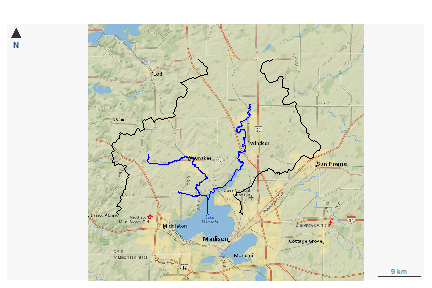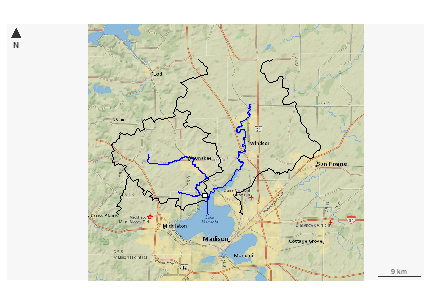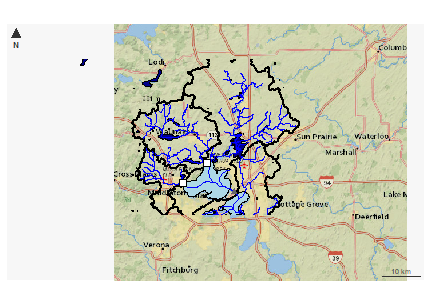# Plotting with nhdplusTools

The goal of this vignette is to demonstrate a simple and lightweight approach to building maps with NHDPlus data.

## The `plot_nhdplus` function

`plot_nhdplus` is a work in progress. Not all inputs in the function have been implemented as of 11/18/2019 and additional functionality will be added later. Please leave feature requests and issues you find in an issue here.

`plot_nhdplus` is a function that makes getting a simple plot of NHDPlus data as easy as possible. It works with other functions from `nhdplusTools` for identifying and retrieving watershed outlet locations. See the `plot_nhdplus` documentation for more info.

If we pass `plot_nhdplus` a single NWIS site id, `nhdplusTools` uses web services to get data and we get a plot like this:

``plot_nhdplus("05428500")``If we want to add other watersheds, we can use any outlet available from the Network Linked Data Index. See “nldi” functions elsewhere in `nhdplusTools`.

``````plot_nhdplus(list(list("nwissite", "USGS-05428500"),
list("huc12pp", "070900020602")))````````````plot_nhdplus(list(list("nwissite", "USGS-05428500"),
list("huc12pp", "070900020602")))``````If we don’t know a site id, we can just pass in one or more latitude / longitude locations.

``````start_point <- sf::st_as_sf(data.frame(x = -89.36, y = 43.09),
coords = c("x", "y"), crs = 4326)

plot_nhdplus(start_point)```````plot_nhdplus` also allows modification of streamorder (if you have data available locally) and styles. This plot request shows how to get a subset of data for a plot and the range of options. See documentation for more details.

``````
source(system.file("extdata/sample_data.R", package = "nhdplusTools"))

plot_nhdplus(list(list("comid", "13293970"),
list("nwissite", "USGS-05428500"),
list("huc12pp", "070900020603"),
list("huc12pp", "070900020602")),
streamorder = 2,
nhdplus_data = sample_data,
plot_config = list(basin = list(lwd = 2),
outlets = list(huc12pp = list(cex = 1.5),
comid = list(col = "green"))))``````We can also plot NHDPlus data without an outlet at all.

``````bbox <- sf::st_bbox(c(xmin = -89.56684, ymin = 42.99816, xmax = -89.24681, ymax = 43.17192),
crs = "+proj=longlat +datum=WGS84 +no_defs")
plot_nhdplus(bbox = bbox)``````